Home Quantum Paradigms Intuitive Models for the Quantum Wave Function Y 1. Historic Roots of Quantum Physics. Quantum physics can trace its roots at least as far back as the mid 18th century. In 1752 Melville showed that an incandescent gas radiates at only discrete wavelengths. This behavior of the relatively isolated atoms or molecules in a gas contrasted mysteriously with the radiation emitted by a solid. In the latter case energy is radiated at all wavelengths. Following Melville’s discovery, it was found that gas atoms/molecules also absorb radiant energy only at discrete wavelengths. Such relatively isolated atoms appear to be oblivious to radiation at other wavelengths. No one could explain why gases should be so selective about emitted and absorbed wavelengths, and a full century slid by. Then in 1859 Kirchoff picked up the thread and showed that, for a collection of same-kind gas atoms (i.e. for a given gaseous element), the emission and absorption wavelengths are identical. Still, the explanation for this selectivity remained a mystery. In 1885 Balmer worked out the first formula for a set of Hydrogen wavelengths. His discovery and similar formulas (subsequently worked out by others) suggested that there must be underlying reasons for such discrete wavelength sets. Yet the reasons remained elusive. As mentioned above, the situation for solids (or for large numbers of atoms bound to one another) was different. For it was known that any solid, at a temperature above absolute zero, radiates energy at all wavelengths (the percentage of total emitted power in a given small range of wavelengths being dependent upon the solid’s temperature). One class of solids of particular interest was the so-called "black bodies." A black body by definition absorbs all of the radiation incident upon it. (And of course it also radiates energy if its temperature remains constant.) In 1899 Lummer and Pringsheim measured the emissive power for black bodies. (The emissive power, R(l,T)dl, is defined to be the power radiated per unit of surface area in wavelength range l to l+dl and at temperature T.) They found that virtually no power is radiated at very short wavelengths. But as one looks at longer and longer wavelengths, the emitted power increases to some maximum. For wavelengths longer than that, the power then falls off again. The curves of emissive power vs. wavelength, obtained by Lummer and Pringsheim (and others), resembled the curves for molecular speeds in a gas (worked out earlier by Maxwell and Boltzmann). In one sense such curves made good sense. Quite as one might expect, the integral (over all wavelengths) of a Lummer-Pringsheim curve … i.e. the total power emitted … is perfectly finite. The theorists went to work attempting to explain the black body emissive spectrum. Rayleigh and Jeans made the plausible assumption that the radiation within a cavity must be standing waves. (The energy density in such a cavity is proportional to the emissive power of the cavity’s walls. Thus the energy density must also be perfectly finite in a cavity.) Rayleigh and Jeans demonstrated mathematically that the number of possible electromagnetic radiation modes per unit volume, in wavelength range l to l+dl, must be n(l) dl = (8p / l4) dl. (1.1) This number presumably correlated with the number of possible dipole oscillator modes in the cavity walls. However, Boltzmann’s work and the equipartition theorem held that energy in a connected system (such as the dipole oscillators in the cavity walls) is equally divided among all of its modes, with kT/2 of energy in each mode. Thus the actual emissive spectrum for black bodies implied some very disquieting results. For in the Rayleigh-Jeans formula (Eq. 1.1) the number of possible modes increases without bound as l approaches zero (or as the radiation moves into the ultraviolet end of the spectrum and beyond). According to classical theory, therefore, it seemed that the cavity energy at any T>0 should be infinite! But this was clearly not the case. This disconnect between classical theory and physical reality was so disconcerting that it was dubbed "the ultraviolet catastrophe!" Several attempts (including that of Rayleigh and Jeans) were made to work out a formula that fit the Lummer-Pringsheim curves. But each of them was successful only over a portion of the curve. In some cases no theoretical explanation accompanied a formula. The formula was simply suggested, a la Balmer, as a mathematical curve-fitting exercise. In 1900 Planck (a) advanced a formula that fit the Lummer-Pringsheim curves, and (b) provided a physical basis for the formula. Like Rayleigh and Jeans, he assumed that the radiation in a black body cavity was absorbed and emitted by vibrating atomic dipoles in the cavity walls. But he further assumed that any given dipole cannot absorb or emit radiation in any amount. Rather he theorized that energy could only be absorbed and emitted in small but finite amounts, dubbed "quanta" (plural for "quantum"). Other considerations indicated that the energy of a quantum must be proportional to the vibrating system’s frequency: E = hn. (1.2) The constant of proportionality (h) in Eq. 1.2 is very tiny, and is called Planck’s constant. The relevance of Planck’s "quantum" hypothesis to the ultraviolet catastrophe was clear: the energy of extremely short wavelength (or extremely high frequency) modes of radiation could only be absorbed and emitted in relatively large amounts … an unlikely scenario for oscillating atomic dipoles. While Planck was busy working out a formula and theoretical basis for black body radiation, Lenard was investigating the manner in which electrons are ejected from an irradiated metallic surface. This so-called photoelectric effect had been known for some time. But in 1900 Lenard reported three rather "unclassical" behaviors. First he found that, when irradiated with light below a certain threshold frequency, a surface ejects no electrons, no matter how intense the radiation is! Secondly, for shorter wavelengths (or higher frequencies) he found that the kinetic energy of ejected electrons increases linearly with the frequency, and does not depend on the intensity of the incident radiation! (Increasing the radiation’s intensity only causes more electrons to be ejected.) Lastly, experimenting with very low intensities, he found that some photoelectrons are ejected practically the instant a metal is irradiated. Classically it might be expected in such low intensity cases that some time might elapse before an electron could absorb enough energy to be ejected. In 1905 Einstein extended Planck’s hypothesis from absorbing/radiating matter to light itself. He suggested that radiation of frequency n interacts with matter in discrete quanta, again of energy E=hn. Lenard’s observations indicated that these interactions occur practically instantaneously … an assumption suggesting that radiant energy is particulate and not spread out continuously in space (as classical Maxwellian theory supposes). In the case of light, the particles were dubbed "photons." Einstein’s insight not only provided a theoretical basis for Lenard’s discoveries, but helped explain subsequent experimental results, including (1) the demonstration (by Franck and Hertz in 1914) that free-flowing electrons give up their kinetic energy, when they collide with gas atoms, only in discrete and finite energy quanta, and (2) Compton’s demonstration (in 1923) that high frequency light scatters loosely bound electrons in a way that can best be described by collision theory among particles. In 1907 Einstein retrenched, extending Planck’s hypothesis about how matter only absorbs/emits radiant energy in quanta to the absorption/emission of heat energy. He thus provided a theoretical basis for the experimentally measured specific heats of various solids. The newly discovered behaviors of nature on atomic scales were beginning to make some sort of sense. Quantum theory and its fundamental tenet, that matter and radiation (and heat and …) interact instantaneously in discrete (if tiny) quanta of energy, was taking shape. In 1913 Bohr used the Planck/Einstein ideas to explain the long-puzzling wavelength spectra of Hydrogen atoms. He used the atomic model suggested by Rutherford, that the Hydrogen atom resembles a tiny star/planet system, with the planet orbiting the much more massive, practically resting star. Of course in the case of an atom, the "planet" is an electron, which carries an electric charge. Now classically a charge going in a circle radiates energy and, lacking a tangential driving force, an atomic electron should quickly lose energy and spiral in toward the nucleus. Furthermore, the emitted radiation should have the same frequency as the orbiting electron. Since atoms don’t collapse to the size of their nuclei but persist indefinitely, and since there were excellent reasons to believe that the Rutherford atomic model was more or less correct, Bohr concluded that classical theory isn’t right in the case of atoms. Orbiting electrons clearly do not radiate constantly. Furthermore, when they do radiate, the frequency is not the electronic orbital frequency called for by Newtonian mechanics. Bohr postulated that the radiation frequency must be proportional to the difference between the energies of the two quasi-stable electron orbitals: n = (Eb – Ea) / h. (1.3) He deduced that in order for this to be so (and for the radiated frequencies to agree with the known discrete spectra for Hydrogen), the electron’s angular momentum around the nucleus can only assume discrete values: L = nh / (2p), n=1,2,… (1.4) There was great excitement about Bohr’s ability to explain so many empirical results. In 1923 deBroglie advanced a sweeping generalization. He suggested that some sort of wave is associated with every particle (whether that particle be a photon of light or a material particle like an electron). And he proposed that the wavelength and frequency of this new kind of wave are related to the particle’s momentum (p) and its energy (E) in the following ways: p = h/l, (1.5) E = hn. (1.6) It turned out that deBroglie didn’t have things quite right. His new kind of wave would prove not to be an attribute of any given particle, but rather to be an attribute of an ensemble of particles (e.g., a very large number of particles with a common momentum). In 1927 Davisson and Germer provided a stunning corroboration of deBroglie’s ideas. In the course of scattering ensembles of electrons off the layers of atoms in a crystal, they discovered that the electrons scatter in particular directions. These directions are fully consistent with the interference of waves being scattered by the crystal’s atomic planes … a phenomenon previously investigated for light by Bragg. Light had of course long been known to be a wave phenomenon. (The new development with light was that it also exhibits particulate behavior.) The discovery that material particles (e.g. electrons) exhibit wave-like behavior took the physics world by storm! Among other things, electrons seem to go where deBroglie’s waves interfere constructively, and to shun those places where destructive interference occurs. Meanwhile, Heisenberg was busy reflecting upon how accurately certain complementary pairs of physical quantities can be determined. He deduced that a particle’s position and momentum (for example) cannot simultaneously be determined with absolute accuracy. Now classically it had always been assumed that such quantities could be perfectly known at some selected instant in time. For example, it was believed that an observer could precisely determine a system’s initial state and, having done this, that the observer could perfectly predict the system’s future states using the classical laws. However, Heisenberg concluded that the more accurate our knowledge of a particle’s position is at some moment, the less accurate our knowledge of its momentum must be (and vice-versa). According to Heisenberg’s Uncertainty Principal the classical assumption, that a system’s initial state can be precisely known at some time t=0, is flawed. In the case of systems containing macroscopic (massive) particles it seems that we can determine the initial states precisely because the uncertainties are relatively insignificant. But rigorously speaking the imprecision is there, and this imprecision becomes more and more significant as the system’s constituent particles become microscopic. All we can say is that the momenta and/or positions of the particles, in an ensemble of "identically prepared" systems, have values within certain ranges. Of course this means that the ensemble of "identically prepared" systems are not truly all identical at t=0, and their states at later times can be expected to be prone to the same uncertainties. Heisenberg, Born and Jordan developed a new kind of mechanics that took these uncertainties into account. Mathematically their theory was based on matrices and is known as matrix mechanics. Meanwhile Schroedinger was pondering the demonstrated wave behavior of microscopic particles. In 1925 he advanced a differential equation for deBroglie’s new "quantum wave function" (dubbed "psi" or Y). The statistical behaviors predicted by this differential equation were quickly dubbed "wave mechanics." One of the distinguishing features of Schroedinger’s Y is that it is complex, with real and imaginary parts. In 1926 Schroedinger demonstrated that the new wave mechanics is equivalent to the matrix mechanics of Heisenberg et al. Dirac subsequently went on to show (in 1930) that matrix mechanics and wave mechanics are two forms of a more general formulation of quantum mechanics. 2. Born’s Interpretation of Y(r,t). While collaborating with Heisenberg and Jordan in the formulation of matrix mechanics, Born was also closely following developments in wave mechanics. Everyone wondered about the nature of the new quantum wave function, Y, and what its value at a given point in space and time might signify. Born was well aware of the fact (courtesy of Davisson and Germer) that particles are detected where quantum waves interfere constructively, and are more rarely detected in regions of destructive interference. Since wave amplitudes can typically assume positive and negative values (and/or since a complex quantity cannot itself map to a real physical quantity) he suggested in 1926 that the amplitude of Y squared (i.e., Y(r,t)Y*(r,t) or |Y(r,t)|2) is proportional to the probability that a particle will be detected in the volume element dV, centered on position r, in the time interval dt, centered on time t.. It is important … very important … to bear in mind that the quantum wave function does not refer to a particular particle. Rather it refers to an ensemble of "identically prepared" systems containing a particular kind of particle (say an electron). In the determinate world of classical physics the focus was usually on a particular system. By applying physical laws, it was believed that the future state of any system could be absolutely predicted, given a precise knowledge of its initial state. But thanks to Heisenberg, it became clear that the initial state of a system cannot be precisely known. More generally, the "identically prepared" systems comprising an ensemble necessarily vary somewhat because of intrinsic uncertainties in pairs of complementary variables (such as position and momentum). Thus it made sense that the positions and momenta of the particles in such an ensemble would vary if the systems were observed at some later time. One can at best only say, a priori, that there is a certain probability a particle will be found in a particular volume element during a particular increment of time, when we examine one of the systems in an ensemble (and similarly for the particle’s momentum). Let us take a closer look at what Born meant by "the probability that a particle will be detected…". We shall consider an ensemble of systems, each containing a single particle (say an electron). We start out at time t=0 with an ensemble of N such "identically prepared" systems, where N is arbitrarily large (or infinite). At some later time t1>0 we look for the particle in each member of an arbitrarily large subset of the ensemble (containing, say, n0. Of course we never actually do things this way! Rather we use quantum theory to determine Y(r,t) and hence P(r,t). Rigorously speaking we would have to perform the above-described experiment for infinite N and n (i.e. over all of space and for all times > 0) in order to exhaustively test our theoretical function Y. It should be borne in mind that P(r,t) may be found to be zero throughout much of space. For example, if we know that our ensemble is initially contained in volume Vinitial, then at times t>0 we can count on the particle not being outside of some expanded, finite volume (assuming the speed of every particle in the ensemble is < c). Outside of that expanded volume we should find P(r,t) to be zero, whether we calculate P a priori (using quantum theory) or actually check all of infinite space out (not likely!). There is a second, somewhat mysterious case where P(r,t)dVdt will turn out to be zero. This occurs within the volumes that can contain the particle at time t>0. Classically we might be inclined to expect that, within any one of these volumes, the particle will be found at least once in every volume element dV (provided only that n is large enough). However, in reality this may not turn out to be the case, owing to the fact that particles may not invariably interact with their environment (and specifically with us) at each and every position in space and time. Let us say that, sometime during the period t to t+dt, we find the particle (somewhere) in m of the systems, where m 0 at all x (i.e., since it is a certainty that we’ll find the particle somewhere every time we look for it), we have the normalization requirement that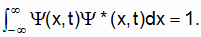(3.15) In view of Eqs. 3.11 and 3.12, Yo must be infinitesimal in the present case. Thus even in some finite interval, Dx, the probability of finding the particle, when we check any given system in the ensemble, is infinitesimal: Y(x,t)Y*(x,t)Dx ~ 0. (3.16) It is only when we look everywhere (i.e. when Dx is infinite) that YY*Dx becomes nonzero (namely unity). 4. Interference. Davisson and Germer were first to demonstrate the effects of interfering deBroglie "matter waves." (G.P.Thomson demonstrated the same thing, using an apparatus patterned after one used by Debye and Scherrer to investigate X-ray diffraction.) An ideal setup would have been one like Young’s double slit experiment with light (performed way back in 1803). But owing to the extremely short wavelengths of "matter waves," no one could at first engineer adequately narrow slits. In 1961 Jonsson solved the problem using minuscule slits in copper foil. Instead of shining a plane electromagnetic wave on the foil (a la Young), however, he used a stream of monoenergetic electrons. On the incident side of the foil this stream approximated a single ensemble, represented by a single wave function. However, when the electrons pass through the slits, two new ensembles are created (say Y1 and Y2), each with its own wave function. These two new wave functions have common wavelengths and frequencies. The beauty of it is that these two new Y waves are in phase at the foil (or at least their phase difference remains constant in time). Owing to the tiny slit widths, each new wave function spreads out in circular wave fronts and the two Y’s begin to interfere beyond the copper screen. Using an array of electron detectors, in a plane parallel to the copper sheet, Jonsson obtained the same patterns of constructive and destructive interference as Young had obtained with light so many years earlier. If one of the slits in the copper sheet is covered, then the interference pattern at the detectors is lost. For example, with both slits open the major peak in the interference pattern typically occurs on a horizontal line midway between the slits and perpendicular to the copper foil. But when one slit is covered this peak diminishes measurably. Indeed one could alternately cover and uncover one of the slits to communicate with the central detector in dot/dash fashion. It is interesting to speculate about how long, after a slit is covered, an attenuation is sensed at the central detector. Since there is a direct connection between the number of electrons sensed per unit time and |Y1 + Y2|2, it is plausible that the time lapse is L/vphase, where L is the distance from either slit to the central detector. Classically we might expect the interference pattern to vanish after a time lapse of L/v. But if vphase=v/2, then wave mechanics indicates that the time lapse will be twice as long!   5. Reflected Y.   Fig. 5.1 depicts the case where a mono-energetic stream of electrons is reversed upon encountering an infinite potential energy barrier at x=0. Figure 5.1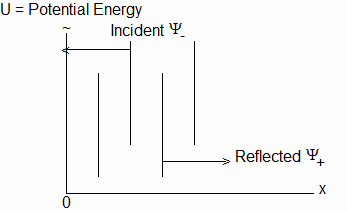Reflected Matter Waves The electrons moving toward negative x constitute one ensemble, and those moving toward positive x constitute another. Each ensemble is characterized by a wave function, say Y- and Y+. It is a simple matter to calculate Y-(x,t) + Y+(x,t), provided one knows what phase shift (if any) occurs at the barrier. In the case of light, there is a phase reversal upon reflection. And indications are that this is also the case with matter waves. Assuming -Y-(0,0) = Y+(0,0) = Yo, where Yo is real and positive, we have Y(0,0) = Y-(0,0) + Y+(0,0) = 0. (5.1) Thus Y(0,0) Y*(0,0) = 0. (5.2) Furthermore, Y-(0,0) and Y+(0,0) both rotate clockwise at the same rate. Thus more generally, Y(0,t) Y*(0,t) = 0. (5.3) Evidently we will never find electrons right at x=0 (although they may be there at certain discrete instants). At values of x other than zero, we have (at time t=0) Y-(x,0) = -Yo[cos(kx) – i sin(kx)], (5.4) Y+(x,0) = Yo[cos(kx) + i sin(kx)]. (5.5) Thus Y(x,0) = Y-(x,0) +Y+(x,0) (5.6) = 2 i Yo sin(kx). Y(x,0) is purely imaginary at all x. It lies all in a plane, and does not spiral around the x-axis. For P(x,0) we have (courtesy of Born) P(x,0) = Y(x,0) Y*(x,0) (5.7) = 4Yo2 sin2(kx). Fig 5.2 illustrates. Figure 5.2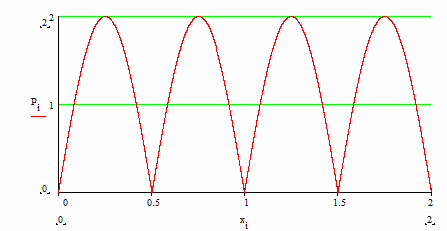P(x), Infinite Barrier at x=0 The probability of finding an electron, which is zero at x=0, rises to a maximum value of 4Yo2 at x=l/4. It then drops back to zero at x=l/2, rises again to 4Yo2 at x=3l/4, etc. This behavior is certainly not what we would classically expect! As in the case of electrons that all move with one common velocity, we might classically expect P(x,0) to be single-valued. But in quantum theory P(x,0) rises and falls as sin2(kx). We do not see this behavior in macroscopic cases because l is so small (typically less than the diameter of an atomic nucleus). It is only when we are dealing with microscopic particles (and longer wavelengths) that the fluctuations in P(x,0) become significant. It is worth pointing out that Y- and Y+ rotate clockwise at every x (quite as they do at x=0). Thus in general, P = 4Yo2 sin2(kx). (5.8) Rigorously speaking, P depends only on x. Such cases are referred to as "stationary states" (i.e., stationary in time). The result that P(x) is not single-valued, but repeatedly rises from zero to a maximum and back again to zero, is the stunning new implication of wave mechanics. It doesn’t have anything to do with interactions between the electrons (or neutrons, or …). Indeed we can let just one electron through to the barrier at a time. After enough have been reflected and subsequently located, we still get the rise and fall in P(r,t). This rise and fall is evidently attributable to the way Y- and Y+ interfere. The ramifications are far-reaching. It was only when physicists had developed techniques for observing nature on a microscopic scale that wave function interference effects became manifestly evident. 6. The Infinite Square Well. In Sect. 5 we could as well have had plane wave Y+ incident on the left side of an infinite barrier. We still would have obtained the sin2(kx) form for |Y- + Y+|2. In Fig. 6.1 we have two infinite barriers, with plane matter waves reflecting off both barriers. This situation corresponds to an ensemble of bound electrons (i.e., bound to the region 0 < x < L). Figure 6.1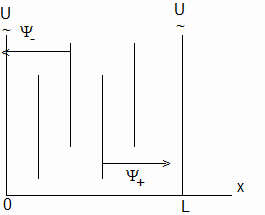Bound Matter Waves In effect, if Y- is propagating to the left and Y+ is propagating to the right, then we again have interference. (We might think of there being a single wave, reversing phase at each reflection and winding around back on itself.) Such setups are well known in the case of electromagnetic waves. And there is an important constraint: the wavelength of the contained radiation must be such that there are electric field nodes at x=0 and x=L. In other words, l must be such that nl / 2 = L, n = 1, 2, … (6.1) Wavelengths that satisfy Eq. 6.1 are said to be resonant. The same constraint holds in the case of matter waves. Thus (according to deBroglie) only discrete particle momenta and energies are resonant. Here again, classically we might expect that a particle of any energy could be contained. However, quantum theory requires resonant momenta/energies. Macroscopically it might seem that a continuum of energies can be bound, owing to the fact that the differences between the resonant energies are too small to detect. In microscopic cases, however, the differences between resonant energies is much more significant, and the fact that only discrete energies can be bound is of fundamental importance. 7. Wave Groups. In previous sections we considered the highly idealized cases where all of the particles in an ensemble of single particles had a single-valued speed (or momentum magnitude). In more realistic situations the particles in a "grand" ensemble of "identically prepared" systems will have a range of momenta. We might do our best always to give the particles the same initial momentum. But the fact that we prepare our systems in some finite volume of space, if nothing else, ensures that the particles’ momenta will take on a spread of initial values. Now the set of particles with any particular momentum magnitude in the range p to p+dp can be considered to be an ensemble in its own right, with its own, simple wave function. This means that the "grand" ensemble’s wave function will be a composite (or sum) of the many simple, constituent wave functions (quite as Y equaled Y- + Y+ in previous sections). Ordinarily the amplitudes of the constituent wave functions will vary as the numbers of particles with different momenta vary. For simplicity, however, let us assume that all of the constituent wave functions have one common amplitude, say unity. In order to sum all of the constituent wave functions, over some range of x and at times t>0, we must know their phase relationships at some x and at initial time t=0. In the case of the infinite potential barrier we opted for Y- to be real at the barrier, at time t=0. And we invoked the phase reversal rule for Y+ at the same position and time. In the case of the square well we made the same assumption. (And the constraint that L = nl/2 gave us the same phase relationship at the other wall.) In the present case, of particles all moving in the positive x-direction with a range of momenta, we shall assume that each constituent Y wave is real and positive at x=0 and t=0. (Other sets of phase relations at x=0 and t=0 may not produce a recognizable wave group in the range of x considered.) Having adopted this rule, the constituent wave functions can be summed at that moment to get the resultant wave function at other values of x. After summing all constituent wave functions to get the resultant Y(x,0), P(x,0) = Y(x,0)Y*(x,0) can be computed. This probability amplitude is plotted in Fig. 7.1. Figure 7.1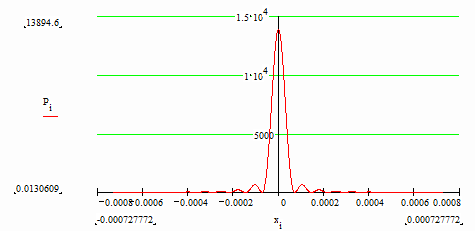P(x,0), Range of Equally Weighted Momenta The perhaps not altogether unexpected result in Fig. 7.1 is that P(x,0) is not a simple gaussian. There are several values of x where P(x,0) goes to zero, but then rises above zero again. Does this mean that there aren’t any particles initially at the "zero" x’s? Or does it mean that any particles at these points won’t interact with their environment (including us)? Of course if our uncertainty in initial particle position is limited to -.0006.0006. The "quantum surprise" is the zero probabilities of finding a particle at certain points in the range -.0006 0 and 0 and 0) drops off with increasing x. It is not unreasonable to suppose that some of the electrons are reflected between 0 and dx, and the rest keep penetrating beyond dx, with some of these being reflected between dx and 2 (dx), etc. Let us assume that the time-independent Schroedinger equation (Eq. 10.4) applies in both Zone 1 and Zone 2. As with the infinite barrier (Sect. 5), in Zone 1 we have y+(1)(x) = y+(1)(0) eikx (11.1) and y-(1)(x) = y-(1)(0) e-ikx. (11.2) Strictly speaking the magnitudes of y+(1)(0) and y-(1)(0) are infinitesimal. But we shall scale them up to finite value yo for practical purposes. In the case of the infinite barrier, we had y+(1)(0) = -y-(1)(0), and thus y(1)(0) and P(1)(0) were zero. However, in the case of a finite barrier P(2)(x) is greater than zero, and it attenuates with increasing x. Therefore P(0) and y(0) must be greater than zero. In order for this to be so, y+(1)(0) and y-(1)(0) cannot be completely out of phase. Fig. 11.2a shows trial values for y+(1)(0) and y-(1)(0). Note that y(1)(0) is completely imaginary for this choice: y(1)(0) = 2i yo sin(q). (11.3) where yo = |y+(1)(0)| = |y-(1)(0)|. Figure 11.2a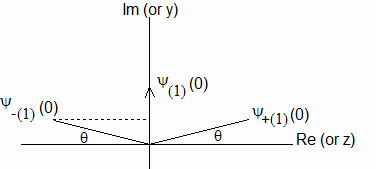Suggested y-(1)(0) and y+(1)(0), Finite Well Now it is clear in Fig. 11.2a that y-(1)(0) = -y+(1)(0) e-2iq. (11.4) Or, since y+(1)(0) = yo eiq, (11.5) it follows that y-(1)(0) = -yo e-iq. (11.6) Substituting in Eq. 11.2, and adding the result to Eq. 11.1, we obtain y(1)(x) = yo (ei(q + kx) – e-i(q + kx)) (11.7) = 2i yo sin(q + k(1)x). Hence P(1)(x) = 4 yo2 sin2(q + k(1)x), (11.8a) dP(1)(x)/dx = (11.8b) 8 yo2 sin(q + k(1)x) k(1) cos(q + k(1)x) = 4 k(1) yo2 sin[2(q + k(1)x)]. Let us now turn our attention to Zone 2. Since no particles are turned back right at x=0, we require that y+(2)(0) y*+(2)(0) = y+(1)(0) y*+(1)(0) (11.9a) and y-(2)(0) y*-(2)(0) = y-(1)(0) y*-(1)(0). (11.9b) The correct way to accomplish this (i.e. the way that doesn’t lead to mathematical impasses) is to specify that y+(2)(0) = - y+(1)(0) (11.10a) and y-(2)(0) = - y-(1)(0). (11.10b)   In other words, y+ and y- undergo complete phase reversals in going from Zone 1 to Zone 2. Fig. 11.2b repeats Fig. 11.2a, with y+(2)(0) and y-(2)(0) included. Figure 11.2b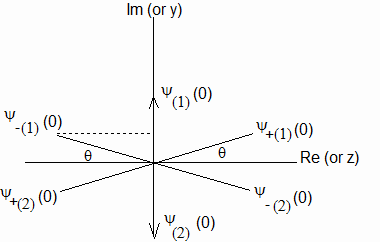y+(0) and y-(0) in Zones 1 and 2 Now since in general mv2/2 = E-U, we see in Zone 2 that mv(2)2/2 is negative: mv(2)2/2 = E-U <0. (11.11) Evidently v(2) is imaginary: v(2) = +i |v(2)|. (11.12) The momentum and wave number are thus also imaginary in Zone 2: mv(2) = +im |v(2)|, (11.13) k(2) = +i 2pm |v(2)| / h. (11.14) Substituting in Eqs. 11.1 and 11.2, we have in Zone 2: y+(2)(x) = y+(2)(0) eix[i2pm|v|/h], (11.15a) y-(2)(x) = y-(2)(0) e-ix[-i2pm|v|/h], (11.15b) where the exponent signs have been chosen so that both y+(2)(x) and y-(2)(x) attenuate in Zone 2. It is clear in Eqs. 11.15a and b that y+(2)(x) and y-(2)(x) do not spiral in Zone 2; they each lie all in a plane: y+(2)(x) = -yo eiqe-ax, (11.16a) y-(2)(x) = yo e-iqe-ax, (11.16b) where a = 2pm|v(2)| / h (11.16c) = 2pm [2(U-E) / m]1/2 / h. Thus y(2)(x) = -2i yo sin(q) e-ax, (11.17a) P(2)(x) = 4 yo2 sin2(q) e-2ax, (11.17b) dP(2)(x)/dx = -8a yo2 sin2(q) e-2ax. (11.17c) Eqs. 11.8a and 11.17b show that P(2)(0) = P(1)(0). (11.18) And requiring that dP(1)(0)/dx (see Eq. 11.8b) equal dP(2)(0)/dx (see Eq. 11.17c) results in sin(2q) / sin2(q) = -2 [(U-E)/E]1/2, (11.19a) or tan(q) = - [E/(U-E)]1/2 (11.19b) and q = tan-1{-[E/(U-E)]1/2}. (11.19c) (Note that q=0 when U is infinite.) The relative size of |y(1)(0)| = |y(2)(0)| is seen in Fig. 11.2b to be |y(1 or 2)(0)| = 2 yo sin(q). (11.20) But y(1)(0) and y(2)(0) are p out of phase. (i.e., like y+(1)(0) and y-(1)(0), y(1)(0) undergoes a full phase reversal on going from Zone 1 to Zone 2.) Rigorously speaking |y(1 or 2)(0)| and yo are infinitesimal in the present case. The physics lies in how q depends on (U-E). Let us summarize: q = tan-1{-[E/(U-E)]1/2}, (11.21a) y(1)(x) = 2i yo sin(q + k(1)x), (11.21b) k(1) = 2p(2mE)1/2 / h (11.21c) y(2)(x) = -2i yo sin(q) e-ax, (11.21d) a = 2p[2m(U-E)]1/2 / h. (11.21e) Fig. 11.3a shows the case for electrons (mass = 9.11E-31 kg), with v(1)=104 m/sec (or E = 4.555E-23 joules) encountering a barrier of height 2E. Fig. 11.3b shows the case for 10-5 kg particles, again with v(1)=104 m/sec (or E = 500 joules) encountering a barrier of height 2E. Note the quantum behavior (i.e. the 3E-8 meter penetration into the forbidden zone) of the microscopic particles in Fig. 11.3a, and the essentially classic behavior (i.e. the 3E-33 meter penetration into the forbidden zone) of the macroscopic particles in Fig. 11.3b. To put these two numbers into perspective, an atom’s diameter is approximately 10-10 meters, and an atomic nuclear diameter is approximately 10-15 meters. Figure 11.3a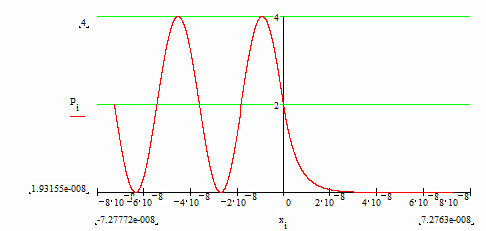Microscopic Particles Penetrating a Finite Barrier Figure 11.3b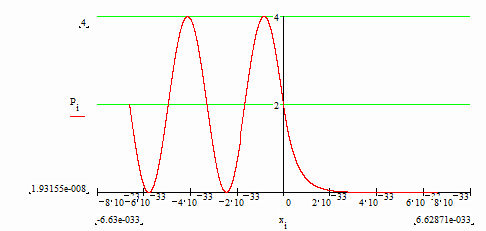Macroscopic Particles Behaving Classically 12. The Finite Square Well. Fig. 12.1 shows a finite square well. Since E = mv(1)2/2 < U, a particle in the well is bound. Classically its momentum would be reversed at x = -L and at x = 0; it would never penetrate into the "forbidden" zones. However, experiment indicates, in the case of microscopic particles, that there is a finite probability of finding the particle at x > 0 (and at x < -L). Figure 12.1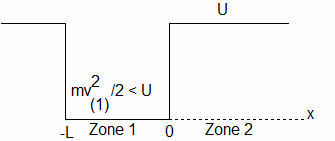A Bound Particle in a Finite Square Well As in the finite barrier case (Sect. 11), P(2)(x) is maximum at x = 0, and falls off with increasing x. Thus here again P(1)(0) and |y(1)(0)| must be greater than zero. q (see Fig. 12.2) depends only on the relative difference between E and U: q = tan-1{-[E/(U-E)]1/2}. (12.1) Figure 12.2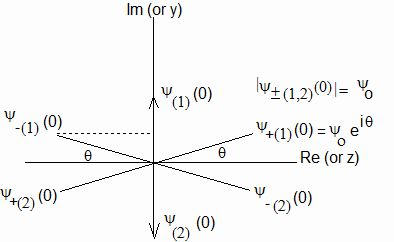y+(1 and 2), Finite Square Well In the finite barrier case E could assume any value. However, in the square well case E is limited to discrete values. In determining the ground state energy for a given relative difference of (U-E)/E, it will be instructive to reconsider the infinite square well. In that case, q (see Eq. 12.1 and Fig. 12.2) is zero. Let us start out with y+(-L) and translate to x=0. We shall suppose that y+(-L) is purely real and positive. As we move along from x = -L to x = 0, the "tip" of y+ moves along a left-handed spiral. At x = 0, y+(0) has a full phase reversal to y-(0) and travels back to x = -L along a right-handed spiral. At x = -L, y-(-L) has another full phase reversal, back to y+(-L), and the process repeats. The longest wavelength (and lowest energy) that fits this scenario satisfies: l/2 = L. (12.2) Fig. 12.3 shows the situation at x = -L and at x = 0 (looking down from "above"). Figure 12.3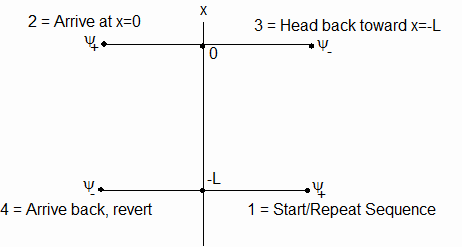y+ at Infinite Square Well Walls Now in Fig. 12.2 we have the same sequence at the finite square well walls. The difference is that y+ does not rotate a full p radians in going from x = -L to x = 0 (or back from x = 0 to x = -L). In the finite well case, y+ rotates only (p - 2|q|) radians. Thus L corresponds to only a partial half-wavelength: L = [(p - 2|q|) / (p)] (l / 2). (12.3) Or, l = [2p / (p - 2|q|)] L. (12.4) And since l = 2p/k, we find (in the ground state) k = (p - 2|q|) / L. (12.5) Furthermore, since E = h2k2 / (8p2m), the finite well ground state energy is: E = h2(p - 2|q|)2 / (8p2mL2). (12.6) Fig. 12.4a plots P(x) for a ground state electron over the range –2L < x < L, using yo = 1 and L = 1E-10. Numerically integrating P(x) over this range and normalizing indicates that the normalized value for yo is yo = 1.987E7. (12.7) Fig. 12.4b plots the normalized P(x). Figure 12.4a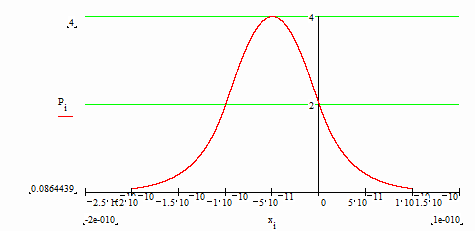P(x), yo Set to Unity Figure 12.4b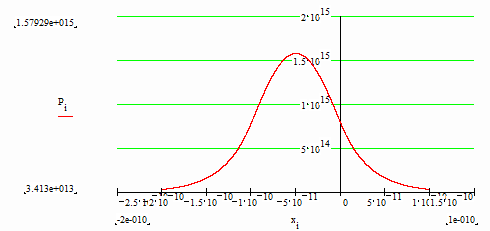Normalized P(x) 13. Tunneling. Fig. 13.1 depicts a stream of monoenergetic electrons, of energy E = mv12/2 < U2, incident upon a potential barrier of finite thickness. Figure 13.1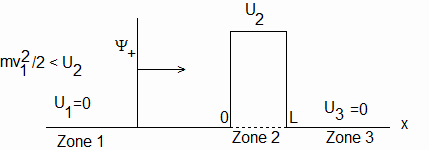A Limited-Extent Barrier Since E